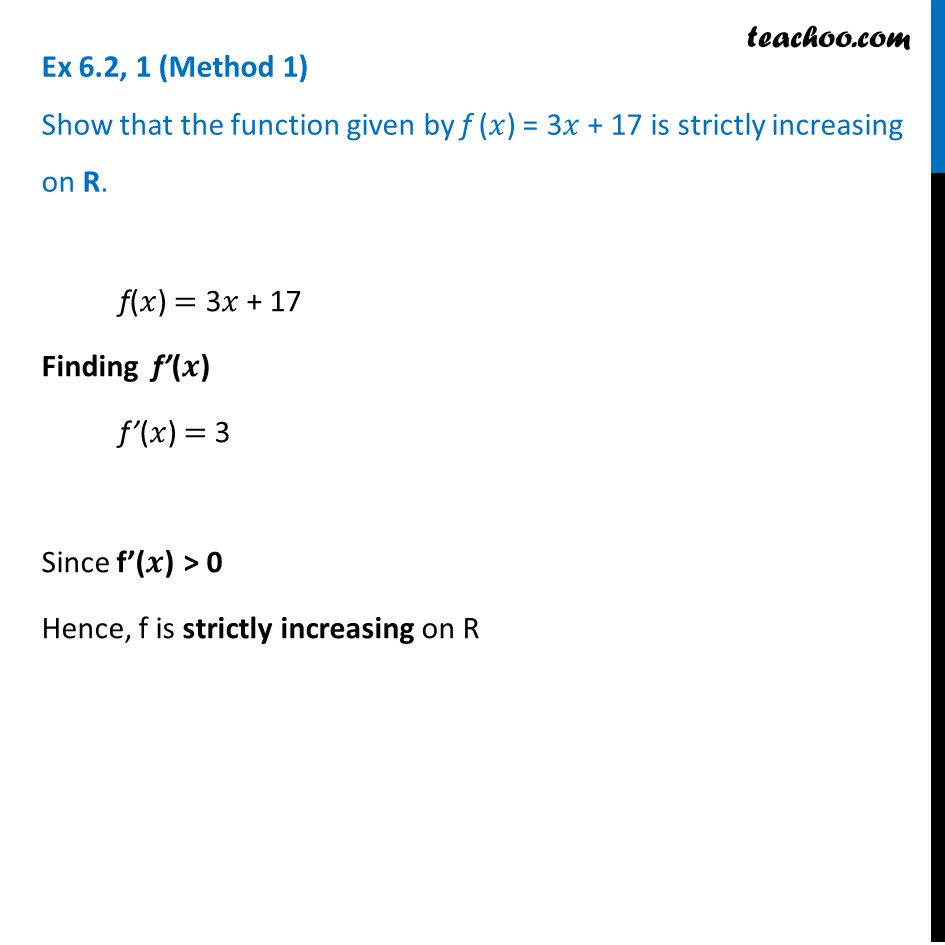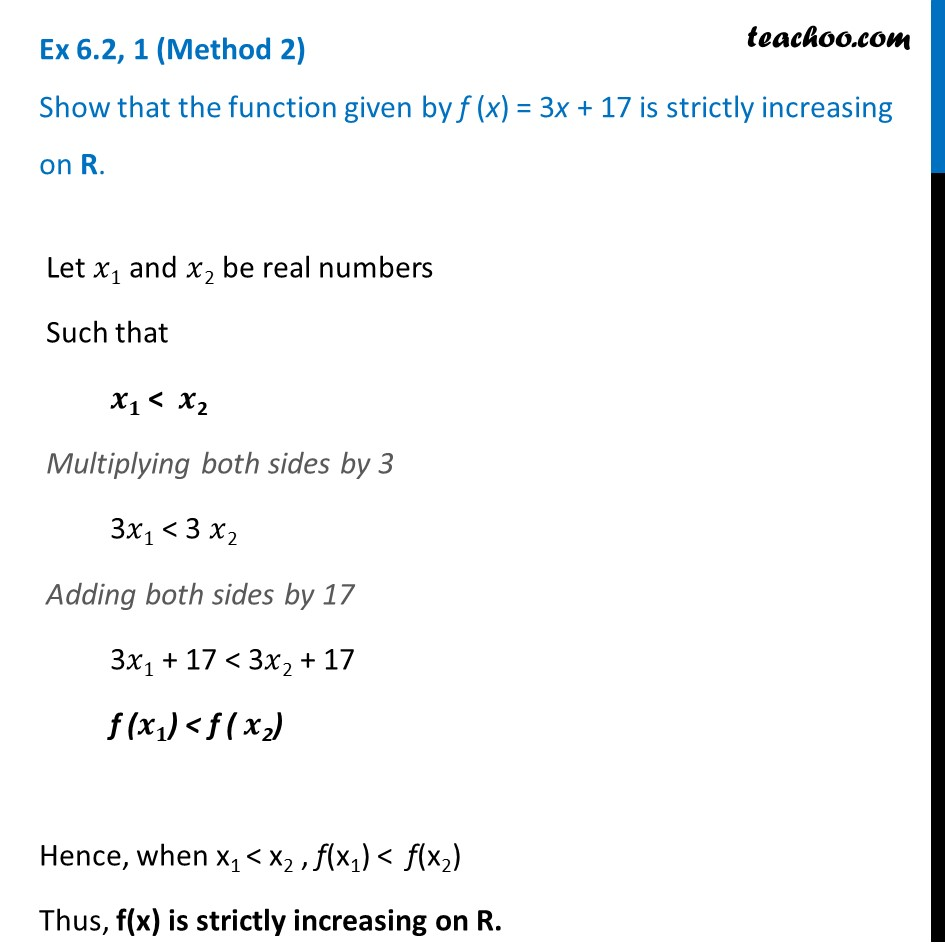Ex 6.2

Chapter 6 Class 12 Application of Derivatives
Serial order wiseLearn in your speed, with individual attention - Teachoo Maths 1-on-1 Class

### Transcript

Ex 6.2, 1 (Method 1) Show that the function given by f (𝑥) = 3𝑥 + 17 is strictly increasing on R. f(𝑥) = 3𝑥 + 17 Finding f’(𝒙) f’(𝑥) = 3 Since f’(𝒙) > 0 Hence, f is strictly increasing on R Ex 6.2, 1 (Method 2) Show that the function given by f (x) = 3x + 17 is strictly increasing on R. Let 𝑥1 and 𝑥2 be real numbers Such that 𝒙𝟏 < 𝒙2 Multiplying both sides by 3 3𝑥1 < 3 𝑥2 Adding both sides by 17 3𝑥1 + 17 < 3𝑥2 + 17 f (𝒙𝟏) < f ( 𝒙2) Hence, when x1 < x2 , f(x1) < f(x2) Thus, f(x) is strictly increasing on R.